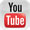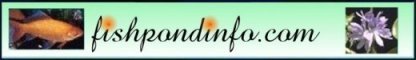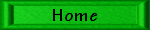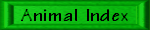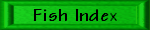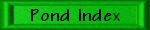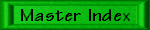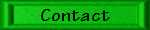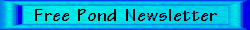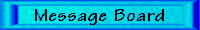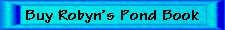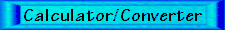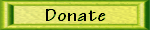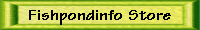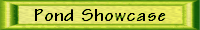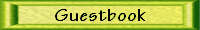Robyn and Roger's Calculator and Converter Page

Last Updated: 7/26/16

Instructions:

My brother is a computer programmer, and he made this Java calculator and converter for you all to use!

The instructions are under construction if that makes sense!

Requirements

Java 1.4.2. plug-in.
Internet Explorer 4 or higher or Netscape 4.x

The calculator will not work on many systems. I am sorry about that. It does not work on my work computer or at home on Netscape (we have an old version), just at home on Internet Explorer.

General Directions

Click one of the top three tabs to get pond/tank volume, unit conversion, or calculator. Then, if you chose one of the first two, you get more choices down the next tab so pick one of those before filling in the actual numbers.

Fill in the boxes with the numbers desired and click on the units to the right to chose the units of the entered numbers. Click units desired for results if applicable and then click the compute button.

Conversions are approximate.

Exponentials

Very large and very small numbers are put into exponential. For example, 1,000,000 is 1 x 106 where the 6 is exponential. However, with this Java calculator, the exponentials are displayed using "E." So, a million may be shown as 1E6. Unfortunately, it does not put spaces in there so you have to be aware and look for the E to indicate exponentials which may not be so obvious if the answer is 1.34586234892734E9 for example (almost didn't notice huh?).

You can enter in numbers exponentially as well using E.

1.0E2 = 1.0 x 10^2 = 100.0

Calculator

Also, note that in the calculator "^" indicates "to the." So, 2^5 is 2 to the fifth or 2*2*2*2*2. Also, 2^0.5 is the square root of 2.

The +, -, *, and / indicate plus, minus, times (multiplication), and divide (division).

History

As you calculate things, the calculator retains previous results in the memory so you can add a few numbers and then go to a conversion or calculation and pull that number up. This is called "history" and can be obtained by clicking on the arrows alongside the entry boxes for numbers.

You can clear the history by clicking "clear history." No matter what though, the number pi or 3.14... will be in there which makes calculating circular circumference or area easy. Here are some pi calculations:

Pond/Tank Volume

The volume of any container can be determined for a rectangle, cylinder (round), or via surface area times depth. If your container, aquarium, or pond is not a perfect rectangle or cylinder, then you divide the body up into multiple pieces and add them together to paste together the need volume.

Available Units

Here are abbreviations for the units available. They are written out in the actual calculator/converter.

Distance: mm, cm, m, km, in, ft, yd, mile, lightyear.
Surface area: all of the above squared plus acre and hectare.
Volume: all of the above cubed plus acre ft, tsp, Tbsp, fl oz, cup, qt, pt, mL, L, US gallon, UK gallon, and bushel.
Temperature: C, F, K.
Weight: mg, g, kg, oz, lb, ton.

All volumes are US fluid unless otherwise noted.

Who Can Use This?

1. Aquarists.
2. Ponders.
3. Cooks.
4. Students.
5. Anyone!

What Can It Do?

1. Add, subtract, multiply, and divide any numbers.
2. Calculate the volume in many units (liter, gallon, etc.) of an aquarium or pond.
3. Convert distances, temperatures, surface areas, weights, and volumes into many units including those commonly used for aquariums, ponds, cooking, and more obscure ones. Ever wonder how many cubic lightyears were in your pond? Find out!

Other web sites with converters for various things:

Online Conversion web site, a generic conversion site with lots of choices (but we have lightyears!).
Chuck Gadd's Planted Aquarium Calculator - this one has neat features for planted aquariums but you have to download it.
Aquarium Volume Calculator - this is good for fast temperature and volume conversions and calculations.
Metric Conversions
The Fish Tank Calculator - this one is useful because it lets you calculate volumes of non-traditional aquariums such as bow front tanks.

Ok, now I am ready! Go now to the calculator/converter!Pet Link Banner Exchange:There have been 51,181,002 file views (file views since 2006, page views before that) to Fishpondinfo from December 1, 2003 through August 17, 2019 (stats lost after that).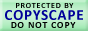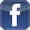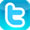E-mail Robyn Copyright © 1997-2019 Robyn Rhudy